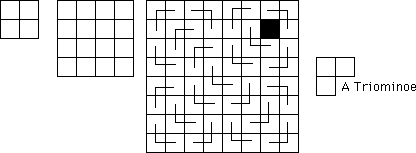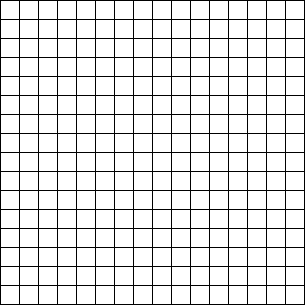# The Technique of Proof by Induction

Suppose that having just learned the product rule for derivatives [i.e. (fg)' = f'g + fg'] you wanted to prove to someone that for every integer n >= 1, the derivative of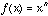is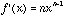. How might you go about doing this? Maybe you would argue like this:

"Well, see that when n=1, f(x) = x and you know that the formula works in this case.

Now for n=2,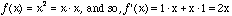Now for n=3,Now for n=4,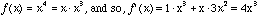Now for n=5,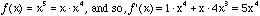And you see we can keep on going this way - do you see the pattern? We just keep using the product rule in conjunction with the result from the previous line and we get the theorem for the next integer."

Consider another example. Suppose that you wanted to show that for every integer n >= 1,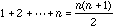. You might argue this way:

It's true for n=1, that's pretty clear. I can also check it directly for n =2, 3 ,4 and 5.

Now that I know it's true for n=5, I can show you that it is true for n=6 like this: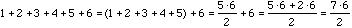and so the formula works for n=6, too. Now I can continue this way and use this last result to show that the formula is true for n=7. Then, using the fact that it is true for 7, show that is also true for 8 etc. I could say something like, "so, see, I can continue just like this and prove the result for one integer after another." But that's not very satisfying.

Now suppose I wanted to prove the following theorem.

Theorem. Suppose that m is a fixed integer and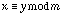.
Then for every integer n >= 1,.

We might recall that we have already shown this is true for n=2 and n=3.

To show that it is true for n=4, we could say that since we know that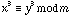and, then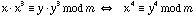. Now that we know that the result is true for n = 4, we can show that it is true for n = 5 in exactly the same way.

Since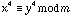and, we have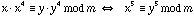Now that we know the result is true for n = 5, we can show that it is true fro n =6 in exactly the same way. Since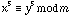and, then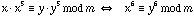. And now we might say that it is fairly evident that we can continue this process and so it is true that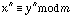for every integer n >= 1.

Mathematical Induction is way of formalizing this kind of proof so that you don't have to say "and so on" or "we keep on going this way" or some such statement. The idea is to show that the result is true for n=1 and then show how once you've shown it to be true for some integer, you can see that it must be true for the next one as well.

## The Principle of Mathematical Induction

Suppose we have an assertion P(n) about the positive integers.

Then if we show both of (i) and (ii) below, then P(n) is true for all n >= 1.

(i). P(1) is true

(ii). For each k >= 1: If P(k) is true, then P(k+1) is true.

So to prove that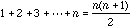we could argue like this:

For n = 1, the result is clearly true since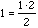.

Now suppose that for integer k >= 1,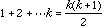. We will be finished if we can show that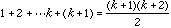. But we have,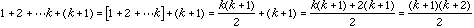and so the result follows by induction.

Similarly,

Theorem. Suppose that m is a fixed integer and.
Then for every integer n >= 1,.

Proof. We will argue by induction. This result is trivial for n=1 it just says that if, then. Hard to argue with that!

Now assume that for some integer k >= 1,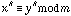. Sinceand since multiplying corresponding sides of congruences is still a congruence,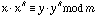. But his last expression reduces to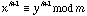. Hence the result follows by induction.

Note: Induction arguments don't always start with the case n = 1. Sometimes we want to prove that an assertion is true for all integers n >= m for some other integer m. In that case we can use the slightly more general version of induction below.

## The Principle of Mathematical Induction

Suppose we have an assertion P(n) about the integers.

Then if we show both of (i) and (ii) below, then P(n) is true for all n >= m.

(i). P(m) is true

(ii). For each k >= m, If P(k) is true, then P(k+1) is true.

Examples

I. The Fibonacci Numbers

The Fibonacci numbers are defined by the recurrence relation,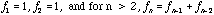So the first few Fibonacci Numbers are:

1, 1, 2, 3, 5, 8, 13, 21, 34, 55, 89, 144, 233, 377, 610,...

There are numerous curious properties of the Fibonacci Numbers. Once guessed, most such properties can be verified by induction. Here are a few examples.

1. For every n >= 1,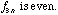.

2. For every n >= 1,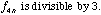3. More generally, For every k >= 1, and n >= 1, if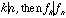.

4. For every n >= 1 and m >= 1,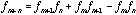.

5. For every n >= 1,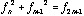.

6. For n >= 2,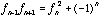.

Another form of Mathematical Induction is the so-called Strong Induction described below.

Principle of Strong Induction

Suppose that P(n) is a statement about the positive integers and

(i). P(1) is true, and

(ii). For each k >= 1, if P(m) is true for all m < k, then P(k) is true.

Then P(n) is true for all integers n >= 1.

We will see examples of this form of induction later in the course.

Also equivalent to the Principle of Induction is the Well-Ordering Principle.

The Well-Ordering Principle simply states that every non-empty subset of the positive integers has a smallest element. This simple observation can serve as the foundation of some very significant mathematical arguments.

Definition. Let n and m be positive integers, and let
A(n,m) = { an + bm >0 : a and b are integers }.

(So, z belongs to A(n, m) if and only if z is an integer, z > 0, and there exists integers a and b such that z = an + bm.)

Theorem. For any positive integers n and m, the smallest element of A(n, m) is gcd(m, n) - the greatest common divisor of n and m.

Proof. By the Well-ordering Principle, A(n, m) does have a smallest element. Let d denote the smallest element of A(n, m). Then d = an + bm for some integers a and b. We will first show that d is a common divisor of m and n. By the divison algorithm, there exist integers q and r with 0 <= r < d and n = qd + r. But then,
r = n - qd = n - q(an + bm) = (qa+1)n - (qb)m.
So if r >0, then r must belong to A(n, m). However, this is impossible since r < d and d is the smallest element of A(n, m). So it must be that r = 0. Hence, n = qd and this means that d divides n. Similarly, d divides m. So we have shown that d is a common divisor of n and m. On the other hand if t is any common divisor of m and n then say n = kt and m = st, then d = an + bm = akt + bst = (ak+bs)t. So d is a multiple of t. Hence d is at least as large as any other common divisor of m and n. Thus d is the largest of the common divisors of n and m. This completes the proof.

Corollary. If n and m are relatively prime, then there exist integers a and b
such that an + bm = 1.

The following simple lemma is often useful. (Its proof is an exercise for you).

Lemma. If n and m are relatively prime, then so are n and n + m, and so are n and n - m.

Exercise: (Let fn denote the nth Fibonacci number.)

1. Show that for each n >= 1, fn and fn+1 are relatively prime.

2. Prove or Disprove: For each n >= 1, fn and fn+2 are relatively prime.

3. Prove or Disprove: For each n >= 1, fn and fn+3 are relatively prime.

# Some Induction Exercises

1. Let Dn denote the number of ways to cover the squares of a 2xn board using plain dominos. Then it is easy to see that D1 = 1, D2= 2, and D3 = 3. Compute a few more values of Dn and guess an expression for the value of Dn and use induction to prove you are right.

2. The triangle inequality says that for any two real numbers x and y,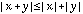.
Show that for any n real numbers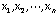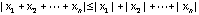3. How many ways are there to cover the squares of a 2xn chessboard with dominos?

Let Dn denote the number of such ways. For example, D1 =1, D2 =2, and D3 =3 as

illustrated below.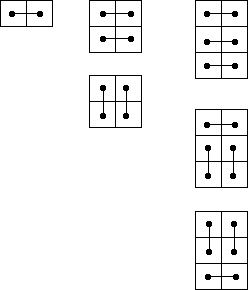Work out the value of D4 and maybe D5, and guess an expression for Dn. To verify your guess you will need to use the strong form of induction. That means that instead of just assuming that the result is true for some k, you assume that it is true for all values less than some k, and then show that it must be true for k.

4. (a). Prove: For n >= 1,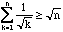.

(b). Does the series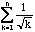converge or diverge?

5. Let an denote the number of subsets of {1, 2, 3, ... n} (including the empty set
and the set itself.)
(a). Show an = 2an-1. (This is pretty simple - you don't need induction here.)
(b). Guess a formula for the value of an and use induction to prove you are right.

6. Prove that for any n>=1, 4 | 3(2n-1) +1

7. Prove by induction: For any n >= 1, 13 + 23 + 33 + ... + n3 = (1 + 2 + 3 + ... + n)2.

Example Induction Proofs

1. Prove that for every n >= 1,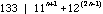We argue by induction. For n=1, the expression has the value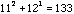. So the assertion is true for n=1.

Now assume that for some integer k,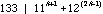. Then there must exist an integer t such that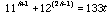. Now we must show that the assertion must be true for k+1, i.e. that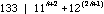.'

However,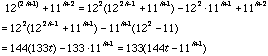So we have, and the result follows by induction.

2. Prove by induction that the sum 1 + 3 + 5 + 7 + ... + 2n-1 is a perfect square.

It is almost impossible to prove this assertion without proving much more.

For convenience, let Sn = 1 + 3 + 5 + 7 + ... + 2n-1. Then Sn+1 = Sn + (2n+1).

Now the result is easily seen to be true in the case k=1, since 1 is a perfect square.

Now assume that Sk is a perfect square, say Sk = t2 for some t. Then we must show that Sk+1 is also a perfect square. Well, Sk+1 = Sk + (2k+1) = t2 + 2k +1.

Now what?? Well, we seem to be stuck. The proof isn't going anywhere.

But look what happens if we try to prove the stronger result that Sn= n2.

Our argument would be almost the same as before except that at the very end

we would have:

Sk+1 = Sk + (2k+1) = k2 + 2k +1 = (k+1)2.

And now the induction proof works!

This is the Inventor's Paradox - it is often easier to prove a stronger result than what you need. Here we prove not just that Sn is a perfect square, but that it is a particular perfect square, namely n2.

3. Let fn be the nth Fibonacci number,
Prove by induction: For every n>=1, 2 | f3n ( i.e. f3n is even)

Proof.
We argue by induction. For n=1 this says that f3 = 2 is even - which it is.

Now suppose that for some k, f3k is even. So f3k = 2m for some integer m.
Now we must show that f3(k+1) is even.

Then, f3(k+1) = f3k+3 = f3k+2 +f3k+1 = f3k+1 + f3k + f3k+1 = 2f3k+1 + f3k = 2(f3k+1 + m ).

4. Prove by Induction: For all integers n >= 1,Proof: For n=1 this asserts that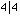- which is certainly true. Now suppose that for some integer k >= 1,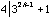. Thus, there is some integer m such that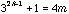.

We claim that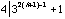. But this is equivalent to showing that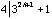.
However,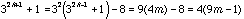, and so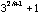is a multiple of 4 and the result follows by induction.

5. Prove by induction: For any n>=1, 7 | 8n - 1.
We argue by induction. For n=1 this just says that 7 | 7 which is true.
Now suppose that for some k>=1, 7 | 8k - 1. So that 8k - 1 = 7t for some integer t.
We must show that 7 | 8k+1 - 1. But, 8k+1 - 1 = 8 ( 8k - 1 ) + 7 = 8(7t) + 7 = 7( 8t +1 ).
And so we have 8k+1 - 1 is a multiple of 7 and so, 7 | 8k+1 - 1.

A Fibonacci Series Problem

Recall that the Fibonacci numbers are defined by the recurrence relation,

fn = fn-1 + fn-2 n>2, f1=1, f2=1.

So, f1=1, f2=1, f3=2, f4=3, f5=5, f6=8, f7=13, f8=21, f9=34, ...

In this exercise we will determine some highly interesting properties of the Fibonacci numbers.

A. Show by induction that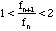for all n>2.

B. Show by induction that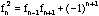.

C. Show (by simple algebraic manipulation) that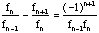.

D. Recall that every bounded, monotone sequence converges. Using this fact, prove:

Lemma. If an is a bounded sequence in which

(i). a3 > a5 > a7 > ...

(ii). a2 < a4 < a6 < ...

(iii). lim |an+1-an|=0

Then an converges.

E. Show that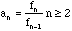satisfies the conditions of the lemma in (D). Consequently, we know that the sequencemust converge to some limit t.

Problem: Find the exact value of t. Note that by (A) your answer should be a number somewhere between 1 and 2.

F. Using the result of (E), determine if the power series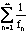converges or diverges.

# More Induction Exercises

1. Show that n lines in general position divide the plane into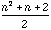regions.

2. If every two cities in state A are joined by a one-way road,then it is possible to find a starting city A and a route from A that passes through every city exactly once.

3. 52n-1 + 1 is divisible by 6.

4. xn-yn is divisible by x-y.

5. n2 + n + 41 is prime for n=1,2...40, but it is not prime for all n >= 1.

6. For every integer n >= 1,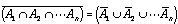holds for any collection of n sets. A1, A2, ..., An.

7. For every integer n >= 1,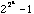is divisible by at least n distinct primes.

8. Show that if x is the root of 1- x - x2 so that x2= 1 - x, then for every integer n >= 1,
x2n = f2n-1 - xf2n.

Example Induction Argument

1. Prove by induction: For all n >= 1, 9n -1 is divisible by 8.

We will argue by induction(1). We first note that for n=1, this just says that 8 | 8 which is clearly true.

Now, assume that the result holds for some(2) integer k. So, 8 | 9k -1, and hence
9k -1 = 8t for some integer t.

We claim that the result is true for the next larger integer, k+1. That is, we claim that

8 | 9k+1 -1. Once we show this we will be finished.

But, 9k+1 -1 = 9( 9k -1 ) + 8 = 9( 8t ) + 8 = 8( 9t +1 ) and so 9k+1 -1 is a multiple of 8, and so 8 | 9k+1 -1. Thus our result follows by induction.(3)

=========================

1. This is just to let everybody know what we are up to so they will know what to expect in the forthcoming argument.

2. Don't say, "assume that the result holds for all n," or anything equivalent to it. That's what you are trying to prove. The word some is significant here.

3. In the algebra at the end don't write:

8 | 9k+1 - 1 = 9( 9k -1 ) + 8

The left-hand side of this expression is an assertion,

"8 divides 9k+1 - 1, " while the right-hand side is an algebraic expression.

Similarly, don't write

8 | 9k+1 - 1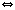9( 9k -1 ) + 8

Either of the following is fine:

9k+1 - 1 = 9( 9k -1 ) + 8 (Both sides are algebraic expressions.)

OR

8 | 9k+1 - 18 | 9( 9k -1 ) + 8 (Both sides are equivalent assertions.)

Induction Problems

1. Prove that for every n >= 1,2. An integer n is a perfect square if it is the square of some other integer.

(For example 1, 4, 9, 16, 25 and 36 are all perfect squares.) Prove by induction that the sum 1 + 3 + 5 + 7 + ... + 2n-1 (i.e. the sum of the first n odd integers) is always a perfect square.

3. Show that any 2n x 2n board with one square deleted can be covered by Triominoes.